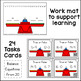Subject
Resource Type
File Type

PDF

(12 MB|44 pages)
Standards
Also included in:
1. Do your students need to practice balancing equations? These task cards are helpful to introduce the concept of balancing equations before balancing equations with a missing addend. The students are to find the sum/difference on both sides of the equal sign. If the answer is the same on both sides,
\$12.00
\$9.00
Save \$3.00
• Product Description
• StandardsNEW

Do your students need to practice balancing equations? These task cards are helpful to introduce the concept of balancing subtraction equations before balancing equations with a missing subtrahend. The students are to find the difference on both sides of the equal sign. If the answer is the same on both sides, then the answer is true. If the answer is different on both sides, then the answer is false. The students can check if the equation is true or false by scanning the QR code. There are practice worksheets as well to support the child's understanding.

*These task cards can be incorporated in small group, math centers, or used as a review.

*Print the desired task card set, laminate, and cut. Place a hole punch in the top left corner of each task card. Keep the cards in order by attaching a ring through the hole punch. Students record their answers on the recording sheet. Enjoy!

Includes:

• 24 Task Cards Balancing Equations Subtracting From 10 (Color & Black/White)
• 24 Task Cards Balancing Equations Subtracting From 20 (Color & Black/White)
• 2 Practice Worksheets Subtracting From 10 (True or False)
• 2 Practice Worksheets Subtracting From 20 (True or False)
• Work mat with a balance scale on it. Students can use cubes to build the number sentence to determine if they have the same number of cubes on both sides. Another option is to solve the subtraction number sentences using the number line located at the top.
Understand the meaning of the equal sign, and determine if equations involving addition and subtraction are true or false. For example, which of the following equations are true and which are false? 6 = 6, 7 = 8 - 1, 5 + 2 = 2 + 5, 4 + 1 = 5 + 2.
Add and subtract within 20, demonstrating fluency for addition and subtraction within 10. Use strategies such as counting on; making ten (e.g., 8 + 6 = 8 + 2 + 4 = 10 + 4 = 14); decomposing a number leading to a ten (e.g., 13 - 4 = 13 - 3 - 1 = 10 - 1 = 9); using the relationship between addition and subtraction (e.g., knowing that 8 + 4 = 12, one knows 12 - 8 = 4); and creating equivalent but easier or known sums (e.g., adding 6 + 7 by creating the known equivalent 6 + 6 + 1 = 12 + 1 = 13).
Total Pages
44 pages
Included
Teaching Duration
N/A
Report this Resource to TpT
Reported resources will be reviewed by our team. Report this resource to let us know if this resource violates TpT’s content guidelines.